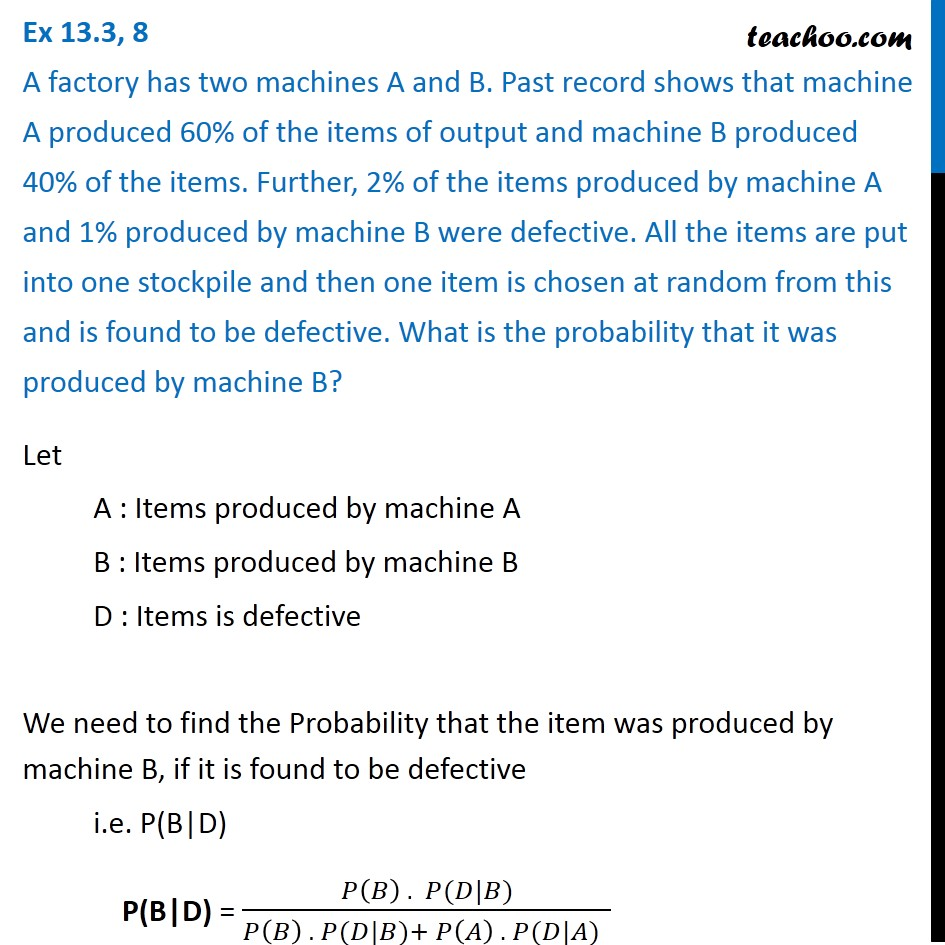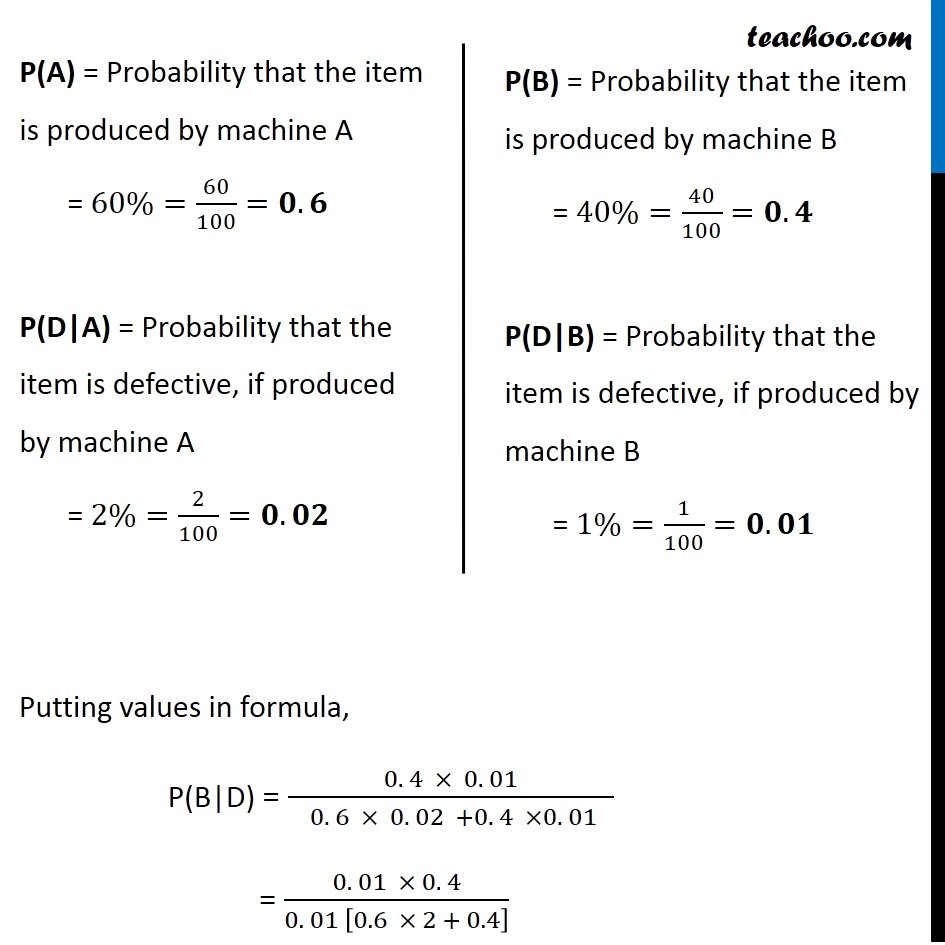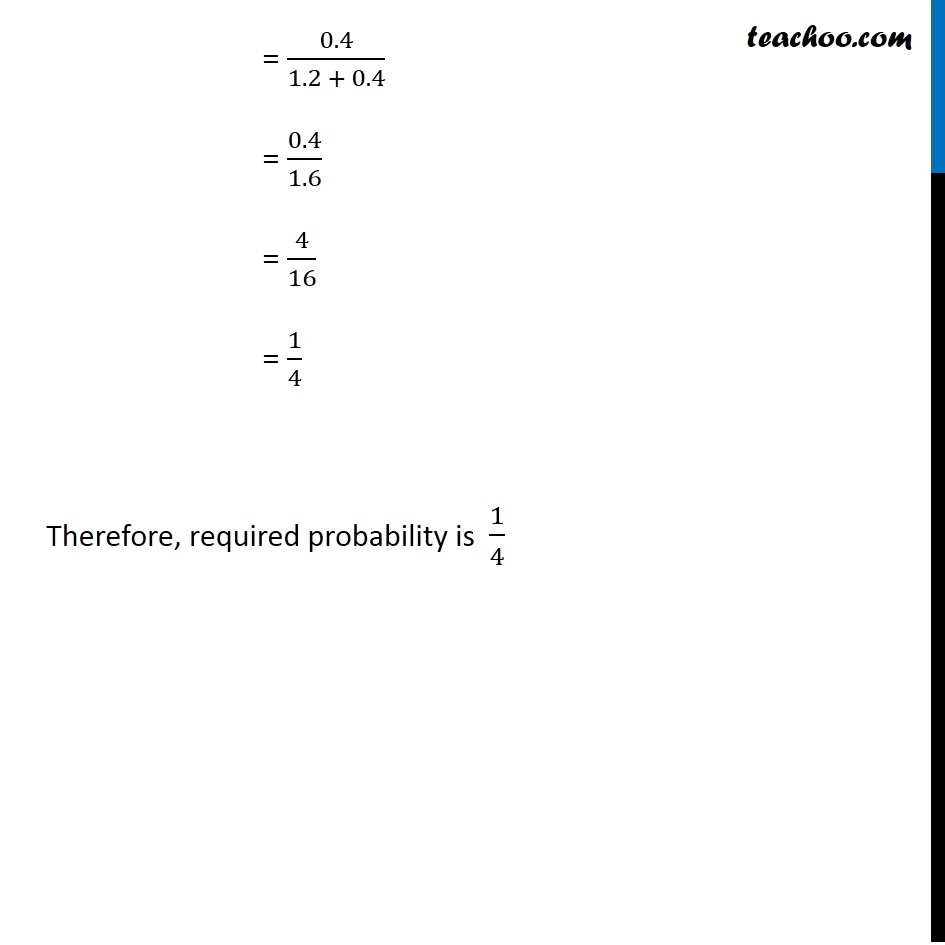Chapter 13 Class 12 Probability

Class 12
Important Questions for exams Class 12Learn in your speed, with individual attention - Teachoo Maths 1-on-1 Class

### Transcript

Ex 13.3, 8 A factory has two machines A and B. Past record shows that machine A produced 60% of the items of output and machine B produced 40% of the items. Further, 2% of the items produced by machine A and 1% produced by machine B were defective. All the items are put into one stockpile and then one item is chosen at random from this and is found to be defective. What is the probability that it was produced by machine B?Let A : Items produced by machine A B : Items produced by machine B D : Items is defective We need to find the Probability that the item was produced by machine B, if it is found to be defective i.e. P(B"|"D) P(B"|"D) = (𝑃(𝐵) . 𝑃(𝐷|𝐵))/(𝑃(𝐵) . 𝑃(𝐷|𝐵)+𝑃(𝐴) . 𝑃(𝐷|𝐴) ) P(A) = Probability that the item is produced by machine A = 60%=60/100=𝟎.𝟔 P(D|A) = Probability that the item is defective, if produced by machine A = 2%=2/100=𝟎.𝟎𝟐 P(B) = Probability that the item is produced by machine B = 40%=40/100=𝟎.𝟒 P(D|B) = Probability that the item is defective, if produced by machine B = 1%=1/100=𝟎.𝟎𝟏 Putting values in formula, "P(B|D)" = (0. 4 × 0. 01)/( 0. 6 × 0. 02 +0. 4 ×0. 01 ) = (0. 01 × 0. 4)/(0. 01 [0.6 × 2 + 0.4] ) = 0.4/(1.2 + 0.4) = 0.4/1.6 = 4/16 = 1/4 Therefore, required probability is 1/4